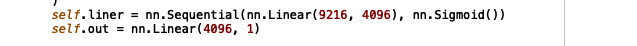# Using model.pth pytorch to predict image

Hello,
I am a beginner in neural networks and I am trying a siamese neural network using Pytorch.

I tried someone’s project that was published on github, but the post only gave me the stage of making a model with the .pth format

how can I make the model can predict the images that I put into the system?

can anyone help me?

Once you have the model and load its `state_dict`, you should set it to evaluation mode (to use the running stats in batchnorm layers and disable dropout). Afterwards, you would have to use the same preprocessing pipeline, which was used during training to get reasonable results (e.g. normalizing with the same mean and stddev). Once you’ve created the input tensors, you could pass them to your model to get the output. Here is a small dummy code snippet:

``````# Create the model
model = MyModel()

# Create the preprocessing transformation here
transform = transforms.ToTensor()

img = Image.open(...)

# Transform
input = transform(img)

# unsqueeze batch dimension, in case you are dealing with a single image
input = input.unsquueeze(0)

# Set model to eval
model.eval()

# Get prediction
output = model(input)
``````
5 Likes

thanks for the help, everything went smoothly until i found this error

can you help me in understanding the error and how can I fix it?Your model apparently needs a second input, which is missing in your call.so how can I use 2 image inputs?

is it enough to make it like this?

sorry if I asked a very basic question.

``````input = transforms(img1)
input = transforms(img2)
input = (img1,img2)
# unsqueeze batch dimension, in case you are dealing with a single image
input = input.unsqueeze(0)

# Set model to eval
model.eval()

# Get prediction
output = model(input)
``````

Just pass two arguments to the model:

``````output = model(x1, x2)
``````
1 Like

i’ve been tried, and i found this error, i feel so confused.the error said that input must be transforms to tensor?
but i think the image has been transformed to tensor by `input=transforms(x1)`

You would have to transform the images (`x1` and `x2`) and pass the tensors to the model.
You are currently reassigning `image` to both transformed tensors.
This should work:

``````x1 = transforms(x1)
x2 = transforms(x2)
output = model(x1, x2)
``````
1 Like

when i try that, then error comes again that said expected image is 4dimension, but my data is 3dimension.
expected is [64,1,10,10] but my dataset is [105, 105, 1]
and i still want to use my own dataset. i search how to solve it and some page said the image must unsequeeze before.

and i see, i dont unsequeeze x1 and x2
but when x1 and x1 pass the unsequezeed, the same error comes again like this

this is my model

``````class Siamese(nn.Module):

def __init__(self):
super(Siamese, self).__init__()
self.conv = nn.Sequential(
nn.Conv2d(1, 64, 10),  # 64@96*96
nn.ReLU(inplace=True),
nn.MaxPool2d(2),  # 64@48*48
nn.Conv2d(64, 128, 7),
nn.ReLU(),    # 128@42*42
nn.MaxPool2d(2),   # 128@21*21
nn.Conv2d(128, 128, 4),
nn.ReLU(), # 128@18*18
nn.MaxPool2d(2), # 128@9*9
nn.Conv2d(128, 256, 4),
nn.ReLU(),   # 256@6*6
)
self.liner = nn.Sequential(nn.Linear(9216, 4096), nn.Sigmoid())
self.out = nn.Linear(4096, 1)

def forward_one(self, x):
x = self.conv(x)
x = x.view(x.size(), -1)
x = self.liner(x)
return x

def forward(self, x1, x2):
out1 = self.forward_one(x1)
out2 = self.forward_one(x2)
dis = torch.abs(out1 - out2)
out = self.out(dis)

return out

``````

when I want to test the resulting model, an error appears stating the requested input is 4 but entering 3 dimensional input.

I think there is a problem in the image that I will test

``````from PIL import Image
x1 = Image.open('t.PNG')
x2 = Image.open('g.PNG')
``````

the error is said that I put an image with a size of 105x105, even though the test image that I input is not 105.

but i was wrong, maybe the error tell me about my data that i use to training.
An image with a size of 105x105 is an image that I used for the train process to produce a siamese neural network model.

I follow this post in the siamese process (https://github.com/Ameyapores/one_shot_learning.git)

That’s an error in your code in line 79,80, with `input` variable. Altought you shouldn’t do a squeeze before go as an input of the model, because model expects a batch dimension

edit: i didn’t notice that it’s an unsqueeze instead of squeeze

i’ve been tried, but still show the same error

``````
x1 = torch.stack([x1])
x2 = torch.stack([x2])
``````

It’s a simple way to add the batch dimension with 1 sample

It’s the same with unsqueeze(0) , I guess that your error was with the lines 79,80 using a wrong variable

Wohooo… its works. Thankyou so much
but when i print the output and just given this score,
How do I tell the label of the score?

This neural network siamese model will predict the image, when the image is the same it will tell the type of the image. for example with a score of -0.005 it is “Table”. correct me if im wrong. hehehe

how to make the output like that? not just giving it a score

Siamese network does not predict the label but the similarity between the inputs. Usually returns a 1 if the images are similar and a -1 if not, but depends on how you trained the network

hmm, oke… thankyou.

but how i can show does it 0 or 1?

I looked the training code and it uses BCEWithLogitsLoss so is using binary cross entropy plus a sigmoid. To get the output between 0 and 1 you have to use a sigmoid like:

`prob = nn.Sigmoid()(output)`

this is the sigmoid model that I used beforemean I have to retrain?